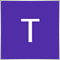# ALGLIB Optimize, Pass parameters to function10

Hello All,

I am trying to use ALGLIB and have managed to simplify a code from TestInterfaces.mqh into the following script given below.

In this case the function is:

`f(x0,x1)=100*(x0+3)^4 + (x1-3)^4 `

How can I pass parameters to a function given by?:

`f(x0,x1)=A*(x0+B)^D + (x1-C)^E `

The documentation says to use CObject &obj, but I don't know how.

```//+------------------------------------------------------------------+
//| Including the libraries                                          |
//+------------------------------------------------------------------+
#include <Math\Alglib\alglib.mqh>
#include <Arrays\ArrayDouble.mqh>

class CNDimensional_Func1 : public CNDimensional_Func
{
public:
CNDimensional_Func1(void);
~CNDimensional_Func1(void);

virtual void      Func(double &x[],double &func,CObject &obj);
};
//+------------------------------------------------------------------+
//| Constructor without parameters                                   |
//+------------------------------------------------------------------+
CNDimensional_Func1::CNDimensional_Func1(void)
{

}
//+------------------------------------------------------------------+
//| Destructor                                                       |
//+------------------------------------------------------------------+
CNDimensional_Func1::~CNDimensional_Func1(void)
{

}
//+------------------------------------------------------------------+
//| This callback calculates f(x0,x1)=100*(x0+3)^4 + (x1-3)^4        |
//+------------------------------------------------------------------+
void CNDimensional_Func1::Func(double &x[],double &func,CObject &obj)
{
func=100*MathPow(x+3,4)+MathPow(x-3,4);
}
//+------------------------------------------------------------------+
//| Script program start function                                    |
//+------------------------------------------------------------------+
void OnStart()
{
double              x[];
double              temparray[];
CObject             obj;

CNDimensional_Func1 ffunc;
CNDimensional_Rep   frep;

CMinLBFGSStateShell state;
CMinLBFGSReportShell rep;

ArrayResize(x,2);
//--- initialization
x=0;
x=0;
double epsg = 1.0e-10;
double epsf = 0;
double epsx = 0;
double diffstep = 1.0e-6;
int maxits = 0;

CAlglib::MinLBFGSCreateF(1,x,diffstep,state);

CAlglib::MinLBFGSSetCond(state, epsg, epsf, epsx, maxits);

CAlglib::MinLBFGSOptimize(state, ffunc, frep, 0, obj);

CAlglib::MinLBFGSResults(state, x, rep);

Print("x = ", DoubleToString(x));
Print("x = ", DoubleToString(x));

}```

Any help is greatly appreciated

Fred309

astaire:

Hello All,

I am trying to use ALGLIB and have managed to simplify a code from TestInterfaces.mqh into the following script given below.

In this case the function is:

How can I pass parameters to a function given by?:

The documentation says to use CObject &obj, but I don't know how.

Any help is greatly appreciated

Fred

Hey Fred, i feel your pain . The support of ALGLIB is completely non existent on MQL5. Metaquotes modified the original ALGLIB source code so as to integrate with MQL5 . The problem is that there is no support for it anywhere on MQL5. You will be lucky if anyone answers . What i keep asking metaquotes is :  Why include a numerical analysis library if you can't even support your modified ALGLIB version ? .

I have been trying a couple of Optimize and integration classes but there is no proper documentation or support anywhere .1654

You have to use a CObject as custom parameter. This is quite a powerful concept, you can pass literally anything as long as it is derived from CObject.

The problem you are facing here is probably that the simpler datatypes like int, double, or arrays thereof, cannot be used right away.

To use a double or int you'd have to define a CNumber or so class that derives from CObject.

For tuples, the CArrayDouble class is suited to hold a double vector and also is a CObject.

So you could just stash your parameters into a CArrayDouble and pass it to the function.

```   CArrayDouble tuple;